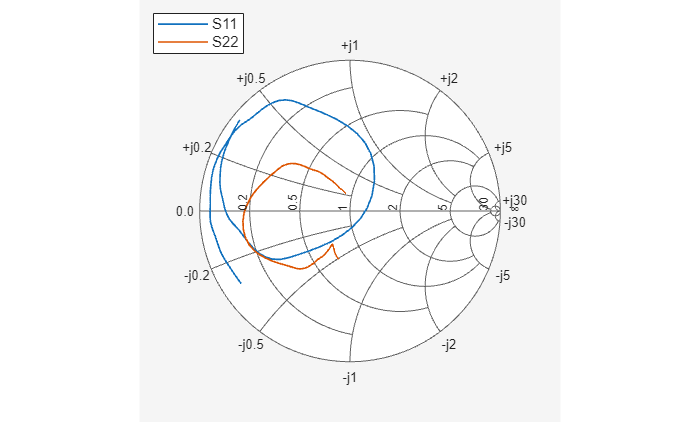In this example, you use the RF Toolbox™ command-line interface to model the gain and noise figure of a cascaded network. You analyze the network in the frequency domain and plot the results.

The network that you use in this example consists of an amplifier and two transmission lines. The toolbox represents RF components and RF networks using RF circuit objects. You learn how to create and manipulate these objects to analyze the cascaded amplifier network.

#### Create RF Components

Type the following set of commands at the MATLAB® prompt to create three circuit (`rfckt`) objects with the default property values. These circuit objects represent the two transmission lines and the amplifier:

```FirstCkt = rfckt.txline; SecondCkt = rfckt.amplifier; ThirdCkt = rfckt.txline;```

#### Specify Component Data

In this part of the example, you specify the following component properties:

• Transmission Line Properties

• Amplifier Properties

#### Transmission Line Properties

Type the following command at the MATLAB prompt to change the line length of the first transmission line, `FirstCkt`, to `12`

`FirstCkt.LineLength = 12;`

Type the following command at the MATLAB prompt to change the line length of the second transmission line, `ThirdCkt`, to `0.025` and to change the phase velocity to `2.0e8`:

```ThirdCkt.LineLength = 0.025; ThirdCkt.PV = 2.0e8;```

#### Amplifier Properties

Type the following command at the MATLAB prompt to import network parameters, noise data, and power data from the `default.amp` file into the amplifier, `SecondCkt`

`read(SecondCkt, 'default.amp');`

Type the following command at the MATLAB prompt to change the interpolation method of the amplifier, `SecondCkt`, to `cubic`

`SecondCkt.IntpType = 'cubic';`

The `IntpType` property tells the toolbox how to interpolate the network parameters, noise data, and power data when you analyze the amplifier at frequencies other than those specified in the file.

#### Validate RF Components

In this part of the example, you plot the network parameters and power data (output power versus input power) to validate the behavior of the amplifier.

Type the following set of commands at the MATLAB prompt to use the `smithplot` command to plot the original S11 and S22 parameters of the amplifier (`SecondCkt`) on a Z Smith® Chart:

```figure lineseries1 = smith(SecondCkt,'S11','S22'); lineseries1(1).LineStyle = '-'; lineseries1(1).LineWidth = 1; lineseries1(2).LineStyle = ':'; lineseries1(2).LineWidth = 1;````legend show`

The plot shows the S-parameters over the frequency range for which network data is specified in the `default.amp` file — from 1 GHz to 2.9 GHz.

Type the following set of commands at the MATLAB prompt to use the RF Toolbox `plot` command to plot the amplifier (`SecondCkt`) output power (Pout) as a function of input power (Pin), both in decibels referenced to one milliwatt (dBm), on an X-Y plane plot:

```figure plot(SecondCkt,'Pout','dBm')````legend show`

The plot shows the power data at 2.1 GHz because this frequency is the one for which power data is specified in the `default.amp` file.

#### Build and Simulate the Network

In this part of the example, you create a circuit object to represent the cascaded amplifier and analyze the object in the frequency domain.

Type the following command at the MATLAB prompt to cascade the three circuit objects to form a new cascaded circuit object, `CascadedCkt`:

```FirstCkt = rfckt.txline; SecondCkt = rfckt.amplifier; ThirdCkt = rfckt.txline; CascadedCkt = rfckt.cascade('Ckts',{FirstCkt,SecondCkt,... ThirdCkt});```

Type the following set of commands at the MATLAB prompt to define the range of frequencies over which to analyze the cascaded circuit, and then run the analysis:

```f = (1.0e9:1e7:2.9e9); analyze(CascadedCkt,f);```

Analyze Simulation Results

In this part of the example, you analyze the results of the simulation by plotting data for the circuit object that represents the cascaded amplifier network.

Type the following set of commands at the MATLAB prompt to use the `smithplot `command to plot the S11 and S22 parameters of the cascaded amplifier network on a Z Smith Chart:

```figure lineseries2 = smith(CascadedCkt,'S11','S22','z'); lineseries2(1).LineStyle ='-'; lineseries2(1).LineWidth =1; lineseries2(2).LineStyle = ':'; lineseries2(2).LineWidth = 1;````legend show`

Type the following set of commands at the MATLAB prompt to use the `plot` command to plot the S21 parameter of the cascaded network, which represents the network gain, on an X-Y plane:`figure`

`plot(CascadedCkt,'S21','dB')``legend show`

Type the following set of commands at the MATLAB prompt to use the `plot` command to create a budget plot of the S21 parameter and the noise figure of the amplifier network:

```figure plot(CascadedCkt,'budget', 'S21','NF')````legend show`

The budget plot shows parameters as a function of frequency by circuit index. Components are indexed based on their position in the network. In this example:

• Circuit index one corresponds to `FirstCkt`.

• Circuit index two corresponds to `SecondCkt`.

• Circuit index three corresponds to `ThirdCkt`.

The curve for each index represents the contributions of the RF components up to and including the component at that index.